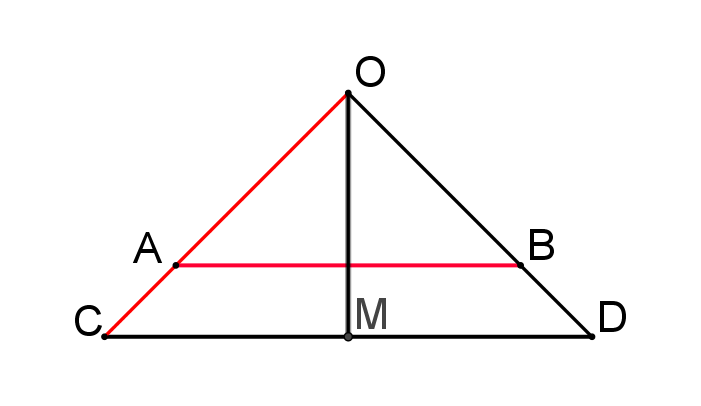#### You may also like### At a Glance

The area of a regular pentagon looks about twice as a big as the pentangle star drawn within it. Is it?### Six Discs

Six circular discs are packed in different-shaped boxes so that the discs touch their neighbours and the sides of the box. Can you put the boxes in order according to the areas of their bases?### Equilateral Areas

ABC and DEF are equilateral triangles of side 3 and 4 respectively. Construct an equilateral triangle whose area is the sum of the area of ABC and DEF.

# Towering Trapeziums

##### Age 14 to 16Challenge Level

Construct the line perpendicular to $CD$ that passes through the midpoint $M$ of $CD$:What do you notice about $\triangle OAB$ and $\triangle OCM?$